# Perpendicular

Determine the slope of the line perpendicular to the line p: y = -x +4.

k =  1

### Step-by-step explanation:

$k=-\frac{1}{-1}=1$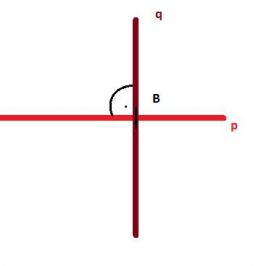Did you find an error or inaccuracy? Feel free to write us. Thank you!Tips to related online calculators
Line slope calculator is helpful for basic calculations in analytic geometry. The coordinates of two points in the plane calculate slope, normal and parametric line equation(s), slope, directional angle, direction vector, the length of the segment, intersections of the coordinate axes, etc.
Do you have a linear equation or system of equations and looking for its solution? Or do you have a quadratic equation?

## Related math problems and questions:

• V - slopeThe slope of the line whose equation is -3x -9 = 0 is
• Perpendicular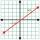What is the slope of the perpendicular bisector of line segment AB if A[9,9] and B[9,-2]?
• Using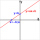Using the point-slope equation, find the equation containing (-7, 3) and slope m = -4
• SlopeWhat is the slope of the line defined by the equation -2x +3y = -1 ?
• SlopeWhat is the slope of a line with an inclination 6.06 rad?
• Slope formFind the equation of a line given the point X(8, 1) and slope -2.8. Arrange your answer in the form y = ax + b, where a, b are the constants.
• LineWrite an equation of a line parallel to To 9x + 3y = 8 That Passes Through The Point (-1, -4). Write in form ax+by=c.
• Slope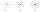Calculate the slope of a line that intersects points (-84,41) and (-76,-32).
• Three points 4The line passed through three points - see table: x y -6 4 -4 3 -2 2 Write line equation in y=mx+b form
• Midpoint 4If the midpoint of a segment is (6,3) and the other endpoint is (8,-4), what is the coordinate of the other end?
• Line segmentFor the line segment whose endpoints are L[-1, 13] and M[18, 2], find the x and y value for the point located 4 over 7 the distance from L to M.
• SlopeFind the slope of the line: x=t and y=1+t.
• Cone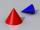If the segment of the line y = -3x +4, that lies in quadrant I, is rotated about the y-axis, a cone is formed. What is the volume of the cone?
• AngleA straight line p given by the equation y = (-8)/(6) x +78. Calculate the size of angle in degrees between line p and y-axis.
• Coordinates of midpointIf the midpoint of the segment is (6,3) and the other end is (8,4) what are the coordinate of the other end?
• Slope RR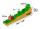A line has a rise of 2 and a run of 11. What is the slope?
• XY triangleDetermine the area of a triangle given by line 7x+8y-69=0 and coordinate axes x and y.